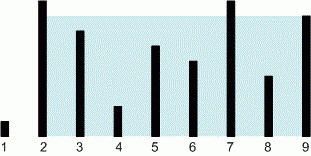Competitions

# Container With Most Water

Time limit 1 second
Memory limit 128 MiB

You are given an integer array height of length n. There are n vertical lines drawn such that the two endpoints of the i-th line are (i, 0) and (i, height[i]).

Find two lines that together with the x-axis form a container, such that the container contains the most water.

## Input data

The first line contains the size n (n10^5) of array height. Second line contains n positive integers - the elements of array height, that are no more than 10^9.

## Output data

Print the maximum amount of water a container can store.## Examples

Input example #1
9
1 8 6 2 5 4 8 3 7

Output example #1
49